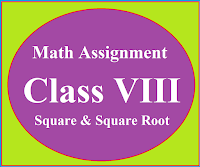### Math Assignment Class VIII | Square & Square RootMath Assignment  Class VIII | Square & Square Root Download or Print free  assignment with answer key  for   Class  8 Squares and  Square Roots.   Important and extra questions that cover all topics of square and square root and is useful and helpful for the students. Math Assignment  Class VIII | Square & Square Root LEVEL -1

## Math Assignment  Class XI  Ch - 13DERIVATIVES

Extra questions of chapter 13 Limits & Derivatives class 11  with answers and  hints to the difficult questions, strictly according to the CBSE and DAV syllabus. Important and useful math. assignment for the students of class 11

## Strictly according to the CBSE and DAV Board

Question 1:  Differentiate the following  by first principal

(i)  sin 2x    (ii)  x ex    (iii)  x2ex    (iv) Cos2x     (v) x2cosx

Question 2 : Using first principle find the derivative of  x sinx

Ans: x cosx + sinx

Question 3 : Find the derivative of sin2x w.r.t. x, by first principle.

Ans: 2sinxcosx = sin2x

Question 4 : Using first principle find the derivative of sin (x2 + 1).

Ans: 2x cos (x2 + 1)

Question 5 : Find the derivative of cos(5x + 2) w.r.t x by first principle.

Ans: - 5sin(5x + 2

Question 6 : Find the derivative of tan(2x + 3) w.r.t. x, by first principle

Ans: 2sec2(2x + 3)

Question 7 : Find the derivative of tan (ax + b) from the first principle.

Ans: asec2(ax + b)

Question 8 : Find the derivative of f(x) =   from first principle.

Ans:

Question 9 : Find the derivative of f(x) =   from first principle.

Ans:

Question 10 : Find the derivative of   using first principal
Ans:
Question 11 : Find the derivative of  f(x) =   with respect to x using first principal.

Ans:

Question 12 : Differentiate :

Ans :

Question 13 : Differentiate:

Ans :    aLoga + axn-1.

Question 14 : Differentiate:

Ans : cosx

Question 15 : Differentiate:

Ans : 3x2tanx + x3sec2x

Question 16 : Differentiate:

Ans : 2x sinx logx + x2 cosx logx + x sinx

Question 17 : Differentiate:

Ans : x4ex (5 + x) + x5(6logx + 1)

Question 18 : Differentiate:
Ans :
Question 19 : Differentiate:
Ans:

Question 20 : Differentiate:

Ans: cosa.sec2x

Question 21 : If y = xsinx + cosx then find    at  x =

Ans: 0

Question 22 : Find the derivative of  cosx - sinx at x =

Ans:

Question 23 : Find the derivative of    w.r.t x

Ans:

Question 24 : Find the derivative of f (x) =  w. r. t. x

Ans:

Question 25 : Differentiate:

Question 26 : Differentiate:

Question 27 : Differentiate:

Question 28 : Differentiate : y =    with respect to x

******************************************

SOLUTION HINT

*****************************************

Formula to find the derivative by using first principal

Product Rule to find the derivative

Quotient rule to find the derivative

Solution Hint Q11

Now by definition of first principle, we have

Applying  CD formula here, we get

Putting the limit in the first cos function we get

Rationalizing the numerator of the angle of sine function, we get

Simplify the above equation we get

Now making the denominator similar to the angle of the sine function, we get

Taking limit and applying the formula  , we get